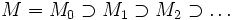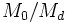# Hilbert-Samuel polynomial

## Definition

Let$A$ be a Noetherian local ring with unique maximal ideal$\mathfrak{m}$. Let$M$ be a finitely generated$A$-module, and$I$ be an ideal of finite colength in$A$ (in other words, there exists a$n$ such that$\mathcal{m}^n \subset I \subset \mathcal{m}$).

Consider an essentially$I$-adic filtration of$M$, i.e. a descending chain:$M = M_0 \supset M_1 \supset M_2 \supset \ldots$

such that there exists$n_0$ such that$M_{n+1} = IM_n$ for$n \ge n_0$.

The Hilbert-Samuel function for this filtration is a function sending a positive integer$d$ to the length of the quotient$M_{d-1}/M_d$ as an$A$-module. This Hilbert-Samuel function turns out to be equal to a polynomial for sufficiently large values of$d$. That polynomial is termed the Hilbert-Samuel polynomial for the filtration.

Some people use the term Hilbert-Samuel polynomial for the length polynomial, which is the polynomial measuring the length of the module$M_0/M_d$.

When we simply talk of the Hilbert-Samuel polynomial, we by default refer to that for the ring as a module over itself. The default ideal is taken to be the maximal ideal and the default filtration is taken to be the standard one.

## Facts

• The specific Hilbert-Samuel polynomial depends on the specific filtration we choose. However, the leading coefficient of the Hilbert-Samuel polynomial depends only on the ideal$I$.
• The leading coefficient depends on the specific choice of the Hilbert-Samuel polynomial. However, the degree is independent of the choice of$I$.
• For a Noetherian local ring$R$, the degree of the Hilbert-Samuel polynomial equals the Krull dimension.